# Multiplication and Division, Part 1

Students begin to explore the concepts of multiplication and division, beginning in the context of equal group and array problems, moving to skip-counting and repeated addition, and ending with more complex and/or abstract problems.

Math

Unit 2

## Unit Summary

Unit 2 opens students’ eyes to some of the most important content students will learn in 3rd grade—multiplication and division. In this unit, "students begin developing these concepts by working with numbers with which they are more familiar, such as 2s, 5s, and 10s, in addition to numbers that are easily skip-counted, such as 3s and 4s," allowing the cognitive demand to be on the meaning of multiplication and division themselves rather than computing (CCSS Toolbox, Sequenced Units for the Common Core State Standards in Mathematics Grade 3). Then in Unit 3, students will work on the more challenging units of 0, 1, 6–9, and multiples of 10.

In 2nd grade, students learned to count objects in arrays using repeated addition (2.OA.4) to gain a foundation for multiplication. They’ve also done extensive work on one- and two-step word problems involving addition and subtraction, having encountered all of the problem types that involve those operations (2.OA.1). Students will rely on this foundational equal-groups understanding and orientation to solving contextual problems extensively in this unit.

At the start of this unit, students gain an understanding of multiplication and division in the context of equal group and array problems in Topic A. To keep the focus on the conceptual understanding of multiplication and division (3.OA.1, 3.OA.2), Topic A does not discuss specific strategies to solve, and thus students may count all objects (a Level 1 strategy) or remember their skip-counting and repeated addition (Level 2 strategies) from 2nd grade to find an unknown product. In Topics B and C, however, the focus turns to developing more efficient strategies for solving multiplication and division, including skip-counting and repeated addition (Level 2 strategies) as well as "just knowing" the facts, which works toward the goal that "by the end of 3rd grade, [students] know from memory all products of two single-digit numbers and related division facts" (3.OA.7). As the Operations and Algebraic Thinking Progression states, "reaching fluency in single-digit multiplications and related division may be quite time consuming because there are no general strategies for multiplying or dividing all single-digit numbers as there are for addition or subtraction" (OA Progression, p. 22). Because "there are many patterns and strategies dependent upon specific numbers," they first work with factors of 2, 5, and 10 in Topic B, since they learned these skip-counting sequences in 2nd grade. Then in Topic C, they work with the new factors of 3 and 4. Only then, when students have developed more familiarity with these factors, will students solve more complex and/or abstract problems with them, including determining the unknown whole number in a multiplication or division equation relating three whole numbers (3.OA.4) and solving two-step word problems using all four operations (3.OA.3, 3.OA.8), assessing the reasonableness of their answers for a variety of problem types in Topic D. Finally, the unit culminates with a focus on categorical data, where students draw and solve problems involving scaled picture graphs and scaled bar graphs, a nice application of the major work of multiplication and division. As the Progressions note, "these developments connect with the emphasis on multiplication in this grade" (MD Progression, p. 7). Students also solve one- and two-step word problems related to the data in these plots, relying on the extensive work students have done with word problems throughout the year. This supporting cluster standard nicely enhances the major work they’ve been working on throughout the unit.

Throughout the unit, students engage in a variety of mathematical practices. The unit pays particular attention to reasoning abstractly and quantitatively, as students come to understand the meaning of multiplication and division and the abstract symbols used to represent them (MP.2). Further, students model with mathematics with these new operations, solving one- and two-step word problems involving them (MP.4).

This introduction to multiplication and division is further deepened in Unit 3, when students will explore the more difficult factors of 0, 1, 6–9, and multiples of 10. Then, in Unit 4, students will explore area and its connections to these operations. In 4th grade, their understanding of multiplication and division will be even more rich, when they will come to understand multiplicative comparison and solve word problems involving it (4.OA.1, 4.OA.2). Further, they’ll solve multi-step word problems involving all four operations, at times needing to interpret a remainder in the context of the problem (4.OA.3). Lastly, students will extend their computation prowess to multi-digit numbers, multiplying a whole number of up to four digits by a one-digit whole number and two two-digit numbers, as well as dividing up to four-digit dividends by a one-digit divisor (4.NBT.5, 4.NBT.6). Because multiplication and division provide a foundation for myriad algebraic and geometric topics from linear functions to trigonometry, this content is critical for all future mathematical learning.

Pacing: 24 instructional days (21 lessons, 2 flex days, 1 assessment day)

Fishtank Plus for Math

Unlock features to optimize your prep time, plan engaging lessons, and monitor student progress.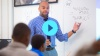## Assessment

The following assessments accompany Unit 2.

### Pre-Unit

Have students complete the Pre-Unit Assessment and Pre-Unit Student Self-Assessment before starting the unit. Use the Pre-Unit Assessment Analysis Guide to identify gaps in foundational understanding and map out a plan for learning acceleration throughout the unit.

### Mid-Unit

Have students complete the Mid-Unit Assessment after lesson 9.

### Post-Unit

Use the resources below to assess student understanding of the unit content and action plan for future units.

Expanded Assessment Package

Use student data to drive your planning with an expanded suite of unit assessments to help gauge students’ facility with foundational skills and concepts, as well as their progress with unit content.

## Unit Prep

### Intellectual Prep

Unit Launch

Prepare to teach this unit by immersing yourself in the standards, big ideas, and connections to prior and future content. Unit Launches include a series of short videos, targeted readings, and opportunities for action planning.#### Intellectual Prep for All Units

• Read and annotate “Unit Summary” and “Essential Understandings” portion of the unit plan.
• Do all the Target Tasks and annotate them with the “Unit Summary” and “Essential Understandings” in mind.
• Take the Post-Unit Assessment.

#### Unit-Specific Intellectual Prep

 Equal groups Example: 4 equal groups of 3 stars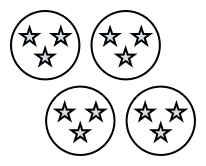Array Example: 4 rows of 3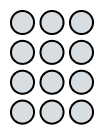One-to-one tape diagram Example: There are 10 teams with 4 students on each team. How many students are there on all of the teams?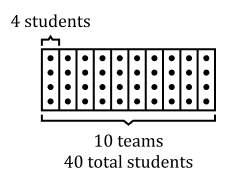Tape diagram Example: There are 4 bags with 3 plums in each bag. How many plums are there in all?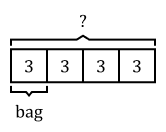Picture graph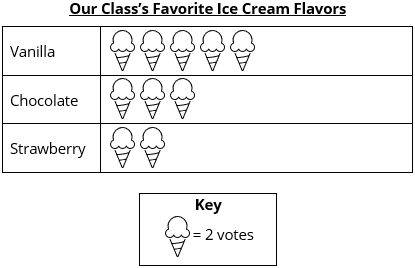Bar graph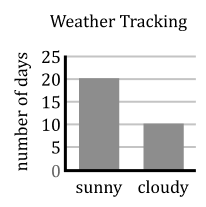### Essential Understandings

• In the United States, the convention for how to think of the equation $$3\times 6 = \square$$ is as 3 groups of 6 things each: 3 sixes (as opposed to 6 groups of 3). "But in other countries the equation 3 × 6 = □ means how many are 3 things taken 6 times (6 groups of 3 things each): six threes. Some students bring this interpretation of multiplication equations into the classroom. So it is useful to discuss the different interpretations and allow students to use whichever is used in their home" (OA Progression, p. 25).
• The equation $$20\div 4 = \square$$ can be interpreted in two ways: there are 20 objects to be partitioned into groups of 4 and we want to know how many groups we can make (the measurement model of division), or there are 20 objects to be partitioned into 4 groups and we want to know how many objects are in each group (the partitive model of division).
• Making sense of problems and persevering in solving them is an important practice when solving word problems. Keywords do not always indicate the correct operation.
• Multiplication problems can be solved using a variety of strategies of increasing complexity, including making and counting all of the quantities involved in a multiplication or division (Level 1 strategy), repeated counting on by a given number (Level 2), and using the properties of operations to compose and decompose unknown facts into known ones (Level 3).

### Materials

• Counters (Maximum of 20 per student or small group) — Students could use a common classroom material, such as paperclips, instead

### Vocabulary

array

division symbol, $${\div}$$

divide/division

dividend

divisor

equal group

factor

key

multiplication symbol, $${\times}$$

multiplication/multiply

product

quotient

row/column

scale

To see all the vocabulary for Unit 2, view our 3rd Grade Vocabulary Glossary.

## Unit Practice

Word Problems and Fluency Activities

Access daily word problem practice and our content-aligned fluency activities created to help students strengthen their application and fluency skills.## Lesson Map

Topic A: The Meaning of Multiplication and Division

Topic B: Multiplication and Division by 2, 5, and 10

Topic C: Multiplication and Division by 3 and 4

Topic D: More Complex Multiplication and Division Problems

Topic E: Scaled Picture and Bar Graphs

## Common Core Standards

Key

Major Cluster

Supporting Cluster

### Core Standards

#### Measurement and Data

• 3.MD.B.3 — Draw a scaled picture graph and a scaled bar graph to represent a data set with several categories. Solve one- and two-step "how many more" and "how many less" problems using information presented in scaled bar graphs. For example, draw a bar graph in which each square in the bar graph might represent 5 pets.

#### Operations and Algebraic Thinking

• 3.OA.A.1 — Interpret products of whole numbers, e.g., interpret 5 × 7 as the total number of objects in 5 groups of 7 objects each. For example, describe a context in which a total number of objects can be expressed as 5 × 7.
• 3.OA.A.2 — Interpret whole-number quotients of whole numbers, e.g., interpret 56 ÷ 8 as the number of objects in each share when 56 objects are partitioned equally into 8 shares, or as a number of shares when 56 objects are partitioned into equal shares of 8 objects each. For example, describe a context in which a number of shares or a number of groups can be expressed as 56 ÷ 8.
• 3.OA.A.3 — Use multiplication and division within 100 to solve word problems in situations involving equal groups, arrays, and measurement quantities, e.g., by using drawings and equations with a symbol for the unknown number to represent the problem.
• 3.OA.A.4 — Determine the unknown whole number in a multiplication or division equation relating three whole numbers. Determine the unknown whole number in a multiplication or division equation relating three whole numbers. For example, determine the unknown number that makes the equation true in each of the equations 8 × ? = 48, 5 = _ ÷ 3, 6 × 6 = ?
• 3.OA.B.5 — Apply properties of operations as strategies to multiply and divide. Students need not use formal terms for these properties. Example: Knowing that 8 × 5 = 40 and 8 × 2 = 16, one can find 8 × 7 as 8 × (5 + 2) = (8 × 5) + (8 × 2) = 40 + 16 = 56. (Distributive property.) Example: If 6 × 4 = 24 is known, then 4 × 6 = 24 is also known (Commutative property of multiplication.) 3 × 5 × 2 can be found by 3 × 5 = 15, then 15 × 2 = 30, or by 5 × 2 = 10, then 3 × 10 = 30. (Associative property of multiplication.)
• 3.OA.B.6 — Understand division as an unknown-factor problem. For example, find 32 ÷ 8 by finding the number that makes 32 when multiplied by 8.
• 3.OA.C.7 — Fluently multiply and divide within 100, using strategies such as the relationship between multiplication and division (e.g., knowing that 8 × 5 = 40, one knows 40 ÷ 5 = 8) or properties of operations. By the end of Grade 3, know from memory all products of two one-digit numbers.
• 3.OA.D.8 — Solve two-step word problems using the four operations. Represent these problems using equations with a letter standing for the unknown quantity. Assess the reasonableness of answers using mental computation and estimation strategies including rounding. This standard is limited to problems posed with whole numbers and having whole-number answers; students should know how to perform operations in the conventional order when there are no parentheses to specify a particular order (Order of Operations).

• 2.MD.D.10

• 2.NBT.A.2
• 3.NBT.A.2

• 2.OA.A.1
• 2.OA.C.3
• 2.OA.C.4

• 4.NBT.B.5
• 4.NBT.B.6

• 4.NF.B.4
• 5.NF.B.3
• 5.NF.B.4
• 5.NF.B.5
• 5.NF.B.6
• 5.NF.B.7

• 4.OA.A.1
• 4.OA.A.2
• 4.OA.A.3
• 4.OA.B.4

### Standards for Mathematical Practice

• CCSS.MATH.PRACTICE.MP1 — Make sense of problems and persevere in solving them.

• CCSS.MATH.PRACTICE.MP2 — Reason abstractly and quantitatively.

• CCSS.MATH.PRACTICE.MP3 — Construct viable arguments and critique the reasoning of others.

• CCSS.MATH.PRACTICE.MP4 — Model with mathematics.

• CCSS.MATH.PRACTICE.MP5 — Use appropriate tools strategically.

• CCSS.MATH.PRACTICE.MP6 — Attend to precision.

• CCSS.MATH.PRACTICE.MP7 — Look for and make use of structure.

• CCSS.MATH.PRACTICE.MP8 — Look for and express regularity in repeated reasoning.

Unit 1

Unit 3

Multiplication and Division, Part 2

## Request a Demo

See all of the features of Fishtank in action and begin the conversation about adoption.

Yes

No

### Any other information you would like to provide about your school?